Get instant live expert help with Excel or Google Sheets“My Excelchat expert helped me in less than 20 minutes, saving me what would have been 5 hours of work!”

#### Post your problem and you’ll get Expert help in seconds.

Your message must be at least 40 characters
Our professional Expert are available now. Your privacy is guaranteed.

# Learn How to Determine Time Intervals in Excel

Time intervals are particularly useful to us especially when we are monitoring or tracking something, in work or at home.  There are two simple ways to determine time intervals in Excel : we can either use AutoFill, or simply do the Math. This step by step tutorial will walk through the process used to determine time intervals in Excel.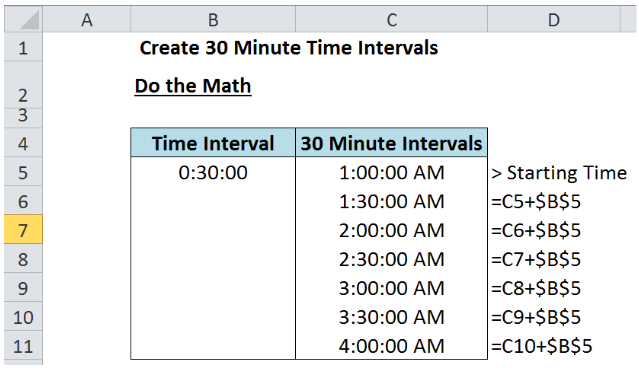Figure 1.  Final result: Determine 30 minute time intervals in Excel

## Setting up the Data

Create the table below and indicate the time interval and starting time.  For this exercise, we will determine 30-minute time intervals. Enter “0:30:00” into cell B5 and “1:00:00 AM” into cell C5.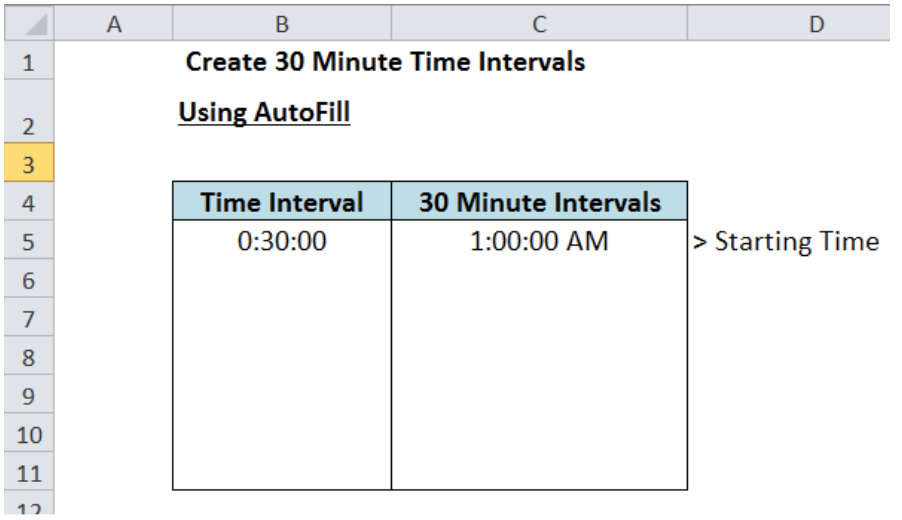Figure 2.  Sample table for determining time intervals using AutoFill in Excel

## Determine Time Intervals Using AutoFill

AutoFill is a feature in Excel that automatically fills cells with data, following a pattern or based on the values of other cells.

• Step 1. In cell C6, enter the formula =C5+B5.

The result is 1:30:00 AM, which is the first 30-minute interval from the starting time of 1:00:00 AM.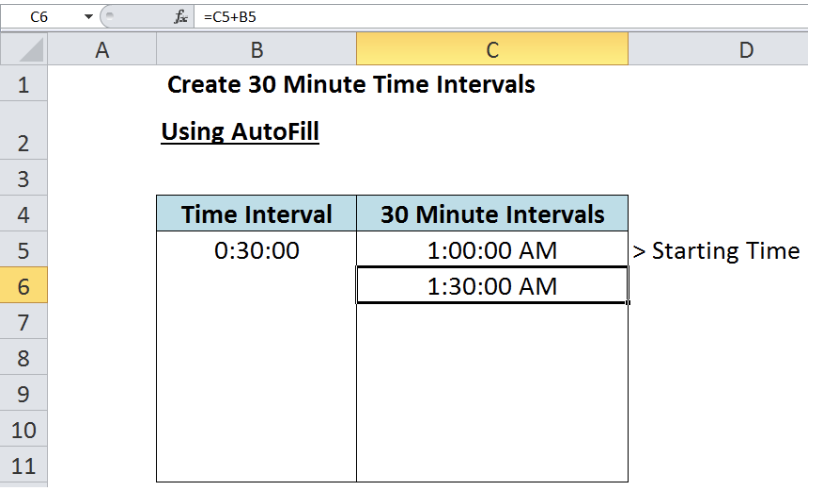Figure 3.  Entering the formula for the first time interval

• Step 2. Highlight cells C5:C6.  These two time intervals will be the pattern that AutoFill will use to fill the other cells with values. Move the cursor to the bottom right corner to turn it into a solid cross.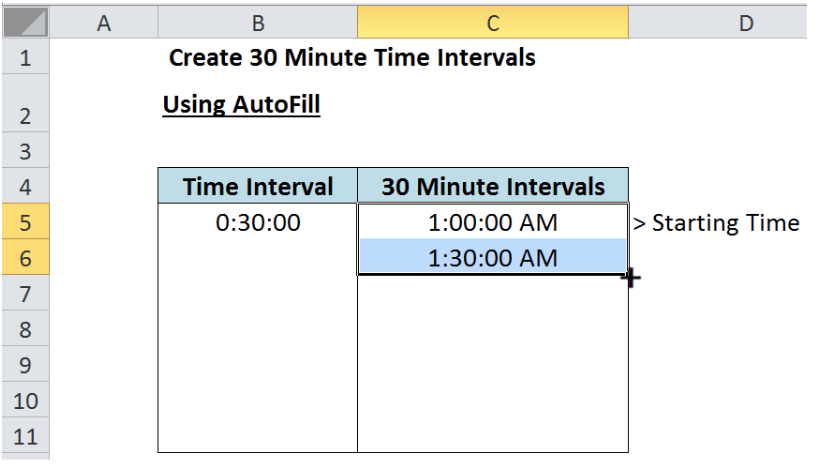Figure 4.  Moving the cursor to the bottom right corner for AutoFill

• Step 3. Drag the cursor down to C11.  We can drag the cursor further down, depending on our desired data for time intervals.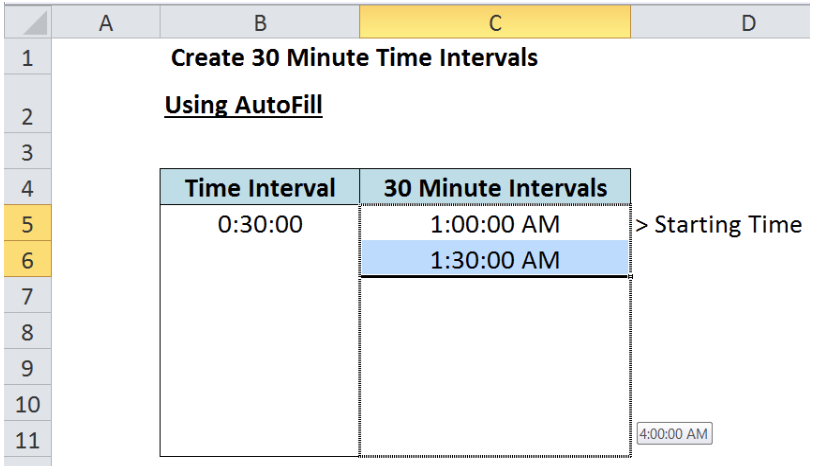Figure 5.  Dragging the cursor for AutoFill

• Step 4. Cells C7:C11 are now filled with the succeeding times, with 30 minutes interval in between.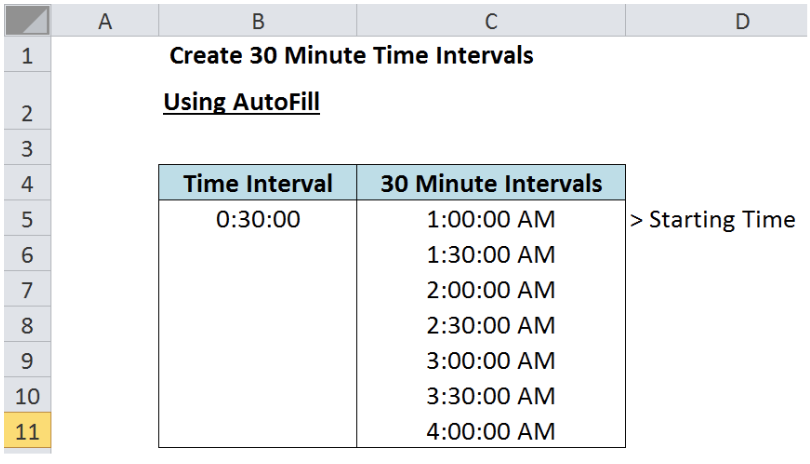Figure 6.  Output: Determine 30 minute time intervals using AutoFill

Note: AutoFill based the filling of values on the first two cells we highlighted, C5 and C6.  Since there is a 30 minutes interval between the two, the other cells are also filled with 30 minutes interval

## Determine Time Intervals by Doing the Math

• Step 1. Set-up the data using a similar table as the previous example.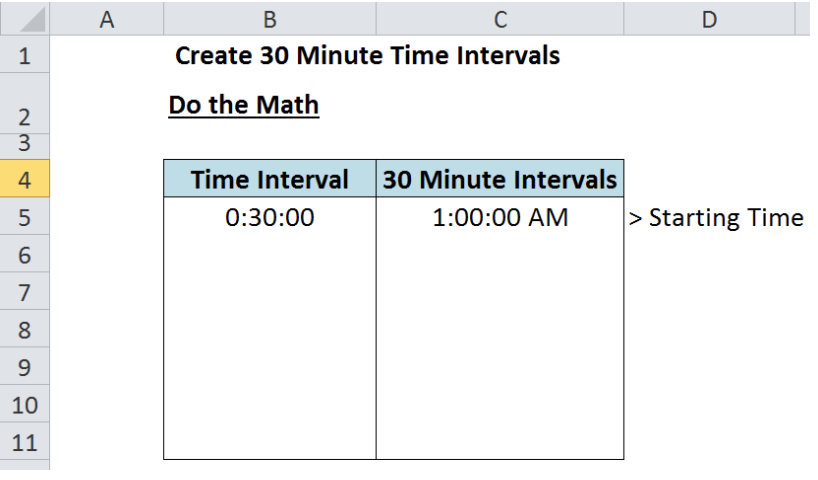Figure 7.  Sample table for determining time intervals in Excel

• Step 2. In cell C6, enter the formula =C5+\$B\$5.  Note that we fix B5 by putting “\$” sign before the column B and the row 5.  This formula adds a constant value “0:30:00” or “30 minutes” to the previous cell.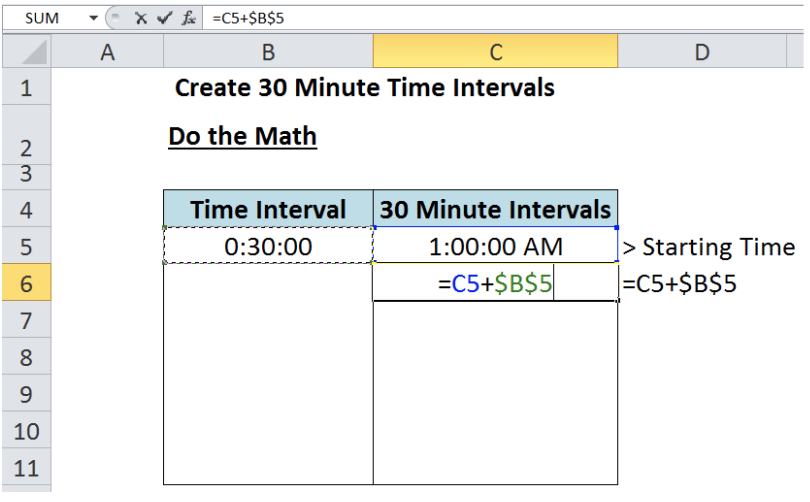Figure 8.  Entering the formula for the first time interval

The result is 1:30:00 AM, which is the first 30-minute interval from the starting time of 1:00:00 AM.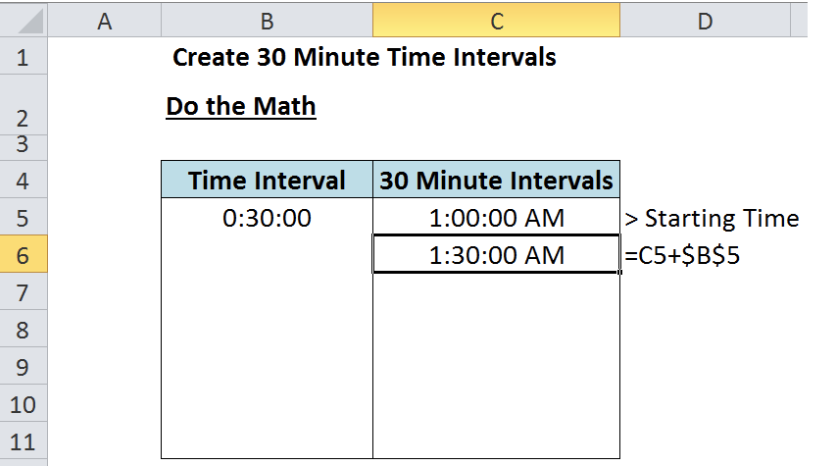Figure 9.  Output for the first time interval

• Step 3. Select C6 and press Ctrl + C to copy it. Select cells C7:C11 and press Enter.  Cells C7:C11 will be filled with the same value as that of C6 which is “1:30:00 AM”.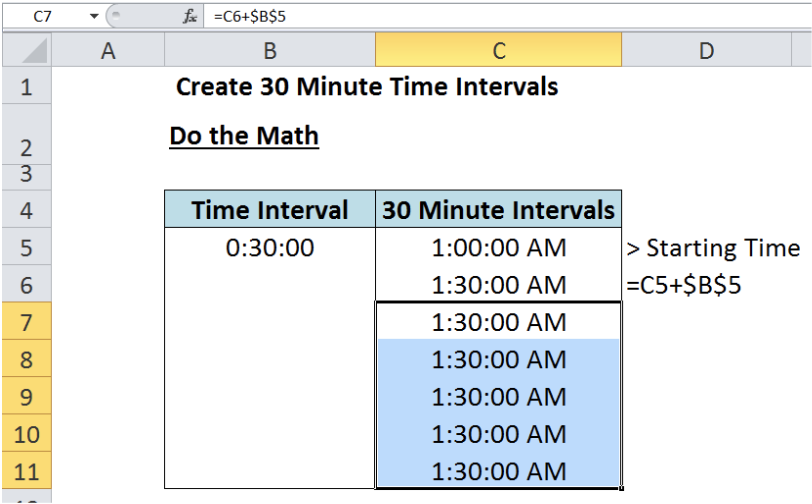Figure 10.  Copying the formula and pasting to the other cells

• Step 4. Press F9 to trigger Excel to calculate and reveal the true values.   Cells C7:C11 are now filled with the succeeding times, with 30 minutes interval in between.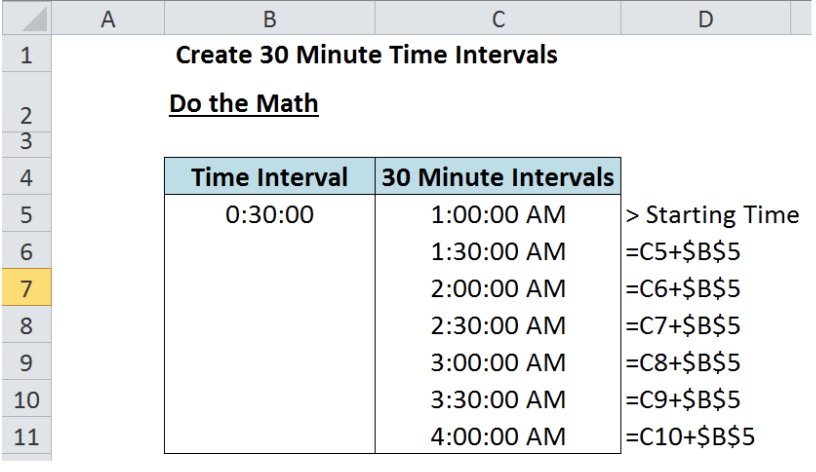Figure 11.  Output: Determine 30 minute time intervals by doing the Math

## More Examples for Determining Time Intervals

By using the template we created, we can determine different time intervals by simply changing the time in cell B5.

Example 1: Determine 60 minutes time interval

For time interval of 60 minutes, enter 0:60:00 into cell B5.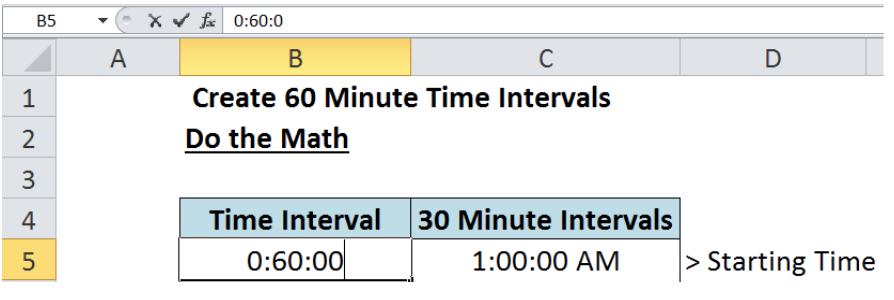Figure 12.  Setting 60 minutes as the time interval

Note:

When we press enter, the value in B5 changes and shows “0.041666667”.  Don’t fret. This is normal. This is the decimal value of one hour in one day, or the decimal value of 1/24.

Excel has a unique way of storing time data and returns a corresponding decimal value, relative to the 24 hours in one day.

See below output for the 60 minute time intervals.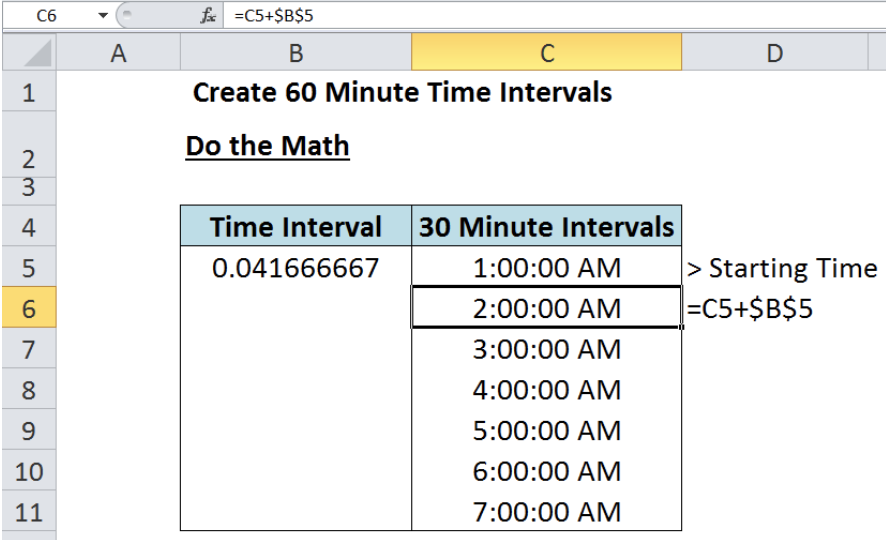Figure 13.  Output: Determine 60 minute time intervals by doing the Math

Example 2: Determine 90 minutes time interval

For time interval of 90 minutes, enter 0:90:00 into cell B5.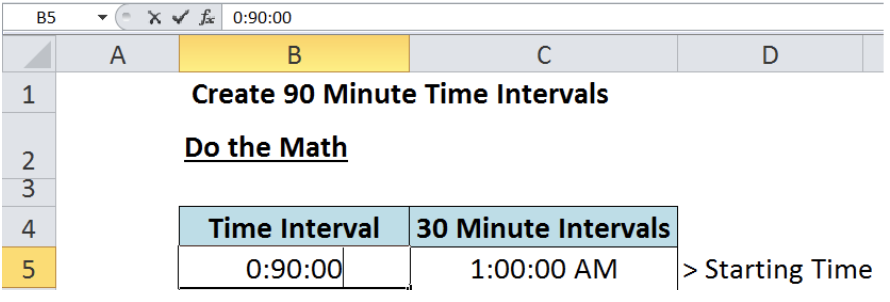Figure 14.  Setting 90 minutes as the time interval

In the same way, when we press enter, the value in B5 changes and returns the decimal value of 90 minutes or 1.5 hours, which is 0.0625. This is the decimal value of 1.5/24, or 1.5 hours in one day.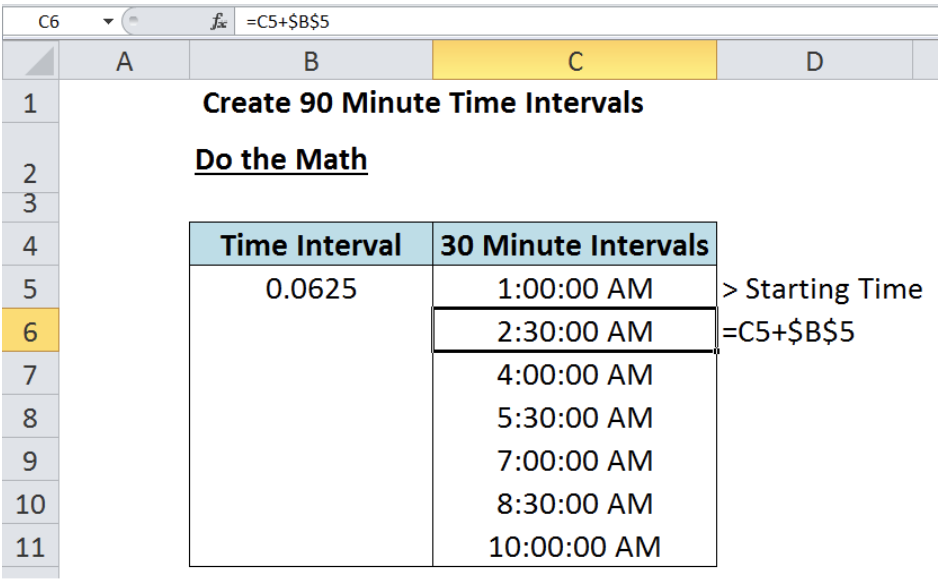Figure 15.  Output: Determine 90 minute time intervals by doing the Math

Most of the time, the problem you will need to solve will be more complex than a simple application of a formula or function. If you want to save hours of research and frustration, try our live Excelchat service! Our Excel Experts are available 24/7 to answer any Excel question you may have. We guarantee a connection within 30 seconds and a customized solution within 20 minutes.

### Did this post not answer your question? Get a solution from connecting with the expert.Another blog reader asked this question today on Excelchat:## Subscribe to Excelchat.coAnother blog reader asked this question today on Excelchat: